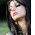Am I Thinking Sufficiently!!

No approach - Low accuracy.
Right approach - 100% accuracy!!

Pointers:

1. DS is not PS. (Most of the times you won't need to get a numerical answer)
2. Get rid of your biases/assumptions. (What's given is what's given and what's not given is not given!!)
3. Memorise the answer options...it's going to be the same on the actual GMAT.
4. When you can't get a definite answer...make an intelligent guess with the help of this:
5. If 1 is sufficient...answer can be A or D. If 2 is sufficient, then answer can be B or D If none is sufficient (alone) then answer can be C or E.
6. Focus on the question stem : Don't loose track of what is being asked.
7. Look at each statement separately. I would suggest you to look at statement 2 first and then statement 1. The reason is that the sequence of the two statements tricks the reader into assuming the info given in 1 and applying it while reading 2. That's wrong!! Most careless errors on DS come when you subconsciously carry over some info from statement 1 to statement 2 (i.e. you're not actually looking at statement 2 on its own).
8. Look at both statements in combination.
9. Use lots of scratch paper. Do it neatly. It'll help.

Given that you follow all the pointers given above...you will start getting most of the DS right now. But I think the most important thing to keep in mind is that tough DS questions will have traps. And you have to be wary if them. Look out for some of these :

1. For example, most people know that if you can set up two linear equations with two unknowns, you can solve for both variables. So the natural instinct when each of the statements can be translated into an equation is to assume that the answer must be C. However, sometimes the two equations may not be independent of each other (e.g. x + 2y = 3 and 2x + 4y = 6, which are just two versions of the same equation).
2. At other times, the question stem may actually ask for a sum (e.g. a+b), and even though there are two variables, a single equation (e.g. 4a + 4b = 20) may be sufficient, since you're only asked to find the sum, not the individual values of a and b.
3. Finally, another common DS trap occurs with Yes/No questions, for example "Is x divisible by 2?". The trick here is that in DS, you are supposed to determine whether the question can be definitively answered based on the given info. So if statement 1 says "X is odd", we can in fact answer the question (in this case, the answer is "no"). So in a Yes/No question, it doesn't matter whether the answer turns out to be Yes or No - all that matters is whether the given info is sufficient to allow you to find the answer.

In addition you would like to pay more attention to the following concepts. These are the toughest concepts, asked on the GMAT.
1. Inequalities...
watch out for points where equality doesnt holds... x > 3 doesnt means x can be 3...
watch out for fractions....higher denominator means lower values....but in negaticve fractions...higher denominator means higher values...

2. squaring a fraction devaluates it...cubing..even more..
but a negative fraction on squaring gets bigger....but on cubing lowers....things like that
3. Modulus...
Learn how mod values are dealt with..
How negative cross multiplications are done with inequalities.
4. Logarithms...
Learn properties of bases...fractional bases, negative values of logs.
I do it with help of graphs.
5. Composite and Prime nos...
clubbed with even and odd concepts..
e.g. 2 is the only no. prime and even, etc.

Cheers!!
P.S. I will put up a flowchart too!!

Suggested Books : Kaplan 800, Kaplan Math Workbook, Official Guide, Manhattan Math

#### You Might Also Like

1.Hi

Just wondering if you have the flowchart in some other section of the website? Could you redirect me to it or place a link on this post please?
Thanks

2.I found th einfo on DS QUITE helpful!

3.nice blog,

but probably need to chnge this on your page.how would a negative fraction lower on cubing it?

-1/3 < -1/9 so on cubing it gets bigger only.

1.Agreed: (-1/3)^3 = -1/27
and
-1/3 = -9/27 < -1/27
so cubing a negative fraction does make it bigger.

4.GMAT Data Sufficiency can be complete only by student interaction.